Test Description

## 10 Questions MCQ Test Mathematics Olympiad for Class 9 | Math Olympiad Test: Quadrilaterals- 1

Math Olympiad Test: Quadrilaterals- 1 for Class 9 2022 is part of Mathematics Olympiad for Class 9 preparation. The Math Olympiad Test: Quadrilaterals- 1 questions and answers have been prepared according to the Class 9 exam syllabus.The Math Olympiad Test: Quadrilaterals- 1 MCQs are made for Class 9 2022 Exam. Find important definitions, questions, notes, meanings, examples, exercises, MCQs and online tests for Math Olympiad Test: Quadrilaterals- 1 below.
Solutions of Math Olympiad Test: Quadrilaterals- 1 questions in English are available as part of our Mathematics Olympiad for Class 9 for Class 9 & Math Olympiad Test: Quadrilaterals- 1 solutions in Hindi for Mathematics Olympiad for Class 9 course. Download more important topics, notes, lectures and mock test series for Class 9 Exam by signing up for free. Attempt Math Olympiad Test: Quadrilaterals- 1 | 10 questions in 10 minutes | Mock test for Class 9 preparation | Free important questions MCQ to study Mathematics Olympiad for Class 9 for Class 9 Exam | Download free PDF with solutions
 1 Crore+ students have signed up on EduRev. Have you?
Math Olympiad Test: Quadrilaterals- 1 - Question 1

### In which of the following figures are the diagonals equal?

Detailed Solution for Math Olympiad Test: Quadrilaterals- 1 - Question 1

Rectangle has equal length of diagonals.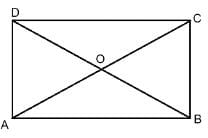Math Olympiad Test: Quadrilaterals- 1 - Question 2

### The angles of a quadrilateral are in the ratio 3 : 5 : 9 : 13. What is the sum of largest and smallest angle of quadrilateral?

Detailed Solution for Math Olympiad Test: Quadrilaterals- 1 - Question 2

Let the angles be 3x, 5x, 9x and 13x
∴ Sum of largest and smallest angle = 3x + 13x = 16x
A/Q,
3x + 5x + 9x + 13x = 360°
⇒ 30x = 360°  ⇒ x = 12
∴ 16x = 16 × 12° = 192°

Math Olympiad Test: Quadrilaterals- 1 - Question 3

### ABCD is a parallelogram in which W, X, Y, Z are mid - points of sides AB, BC, CD and DA respectively. AC is the diagonal, then which of the following is correct?

Detailed Solution for Math Olympiad Test: Quadrilaterals- 1 - Question 3

In ΔACD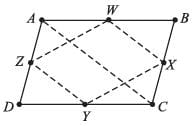ZY ∥ AC and YZ = 1/2 [Using mid-point theorem]

Math Olympiad Test: Quadrilaterals- 1 - Question 4

If consecutive sides of a parallelogram are equal then it is a (none of the angle ≠ 90°)

Detailed Solution for Math Olympiad Test: Quadrilaterals- 1 - Question 4

Rhombus is a parallelogram having consecutive sides equal and none of the angles equal a right angle.

Math Olympiad Test: Quadrilaterals- 1 - Question 5

The diagonals of a rectangle PQRS meet at O. If ∠QOR = 44° Then what is the measure of ∠OPS?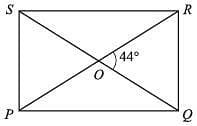Detailed Solution for Math Olympiad Test: Quadrilaterals- 1 - Question 5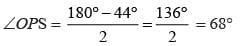Math Olympiad Test: Quadrilaterals- 1 - Question 6

In ΔABC, AD is the median through A and E is the mid-point of AD. BE produced meets AC in F. Then which of the following is correct?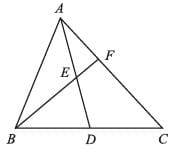Detailed Solution for Math Olympiad Test: Quadrilaterals- 1 - Question 6

∵ AD is the median of ΔABC
∴ BD = DC
Through D, draw DR || BF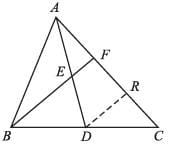Now, in ΔBFC,
DR || BF and D is the mid-point of BC
∴ R should be the mid-point of FC (according to converse of mid-point theorem)
∴ FR = RC ...(i)
E is the mid-point of AD and EF || DR
∴ F should be the mid-point of AR
∴ FR = AF ...(ii)
Using (i) and (ii)
FR = RC = AF
⇒ AC = 3AF
⇒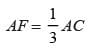Math Olympiad Test: Quadrilaterals- 1 - Question 7

The resulting figure obtained from joining the consecutive mid points of side of a square is

Detailed Solution for Math Olympiad Test: Quadrilaterals- 1 - Question 7

The resulting figure will be a square

Math Olympiad Test: Quadrilaterals- 1 - Question 8

Select the incorrect statement.

Detailed Solution for Math Olympiad Test: Quadrilaterals- 1 - Question 8

A kite is generally not considered a parallelogram because a kite is a quadrilateral whose four sides can be grouped into two pairs of sides of the same length that are adjacent to each other.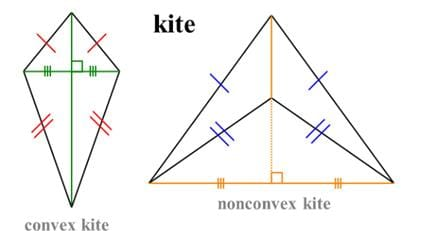Math Olympiad Test: Quadrilaterals- 1 - Question 9

ABCD is a parallelogram and X, Y are the mid-points of sides AB and CD respectively, then

Detailed Solution for Math Olympiad Test: Quadrilaterals- 1 - Question 9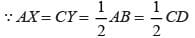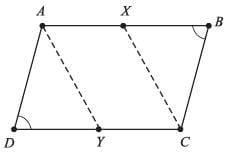And also,
AB || CD, or AX || CY
∵ AX = CY and AX || CY
∴ AXCY is a parallelogram
(∴ one pair of sides are equal and parallel)

Math Olympiad Test: Quadrilaterals- 1 - Question 10

The line segment joining the mid -points of the diagonals of a trapezium is

Detailed Solution for Math Olympiad Test: Quadrilaterals- 1 - Question 10

P and Q are the mid-points of BD and AC respectively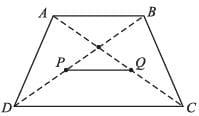Applying mid-point theorem
PQ ∥ AB ∥ DC, and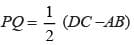## Mathematics Olympiad for Class 9

1 videos|43 tests
 Use Code STAYHOME200 and get INR 200 additional OFF Use Coupon Code